# A Correlogram tale

In data analysis, we usually start with the descriptive statistical properties of the sample data (e.g. mean, standard deviation, skew, kurtosis, empirical distribution, etc.). These calculations are certainly useful, but they do not account for the order of the observations in the sample data.

Time series analysis demands that we pay attention to order, and thus requires a different type of descriptive statistics: time series descriptive statistics, or simply correlogram analysis. The correlogram analysis examines the time-spatial dependency within the sample data and focuses on the empirical auto-covariance, auto-correlation, and related statistical tests. Finally, the correlogram is a cornerstone for identifying the model and model order(s).

What does a plot for autocorrelation (ACF) and/or partial autocorrelation (PACF) tell us about the underlying process dynamics?

This tutorial is a bit more theoretical than prior tutorials in the same series, but we will do our best to drive the intuitions home for you.

## Background

First, we’ll start with a definition for the auto-correlation function, simplify it, and investigate the theoretical ACF for an ARMA-type of process.

## Auto-correlation function (ACF)

By definition, the auto correlation for lag k is expressed as follows:

$$ACF(k)=\rho _k=\frac{\gamma _k}{\gamma_0}$$ $$\gamma _k=E\left [ (x_t-\mu)(x_{t-k}-\mu_{t-k}) \right ]=E\left [ x_{t-k}x_t \right ]-\mu ^2$$ $$\gamma _0=E\left [ (x_t-\mu )^2 \right ]=E\left [ x_t^2 \right ]-\mu ^2$$

Where

• $\rho _k=$ auto-correlation function for lag k
• $\gamma _k=$ auto-covariance for lag k
• $\gamma _0=\sigma ^2=$ time series variance (un-conditional)
• $\mu_{t-k}=\mu_t=\mu=$ stationary time series unconditional mean

Furthermore, let’s assume that ${x_t}$ is generated from a weak-stationary process with a zero mean (i.e.$\mu=0$).

$$\rho _k=\rho_{-k}=\frac{E\left [ x_tx_{t-k} \right ]}{E\left [ x_t^2 \right ]}=\frac{E\left [ x_tx_{t-k} \right ]}{\sigma ^2}$$

For finite sample data, the empirical auto-correlation is expressed as follows:

$$\hat{\rho }_k=\frac{\sum_{t=k}^{N}(x_t-\bar{x})(x_{t-k}-\bar{x})}{\sum_{t=k}^{N}(x_t-\bar{x})^2}=\frac{\sum_{t=k}^{N}(x_{t-k}x_t)-(N-k)\bar{x}^2}{\sum_{t=k}^{N}x_t^2-(N-k)\bar{x}^2}$$

And

$$\hat{\rho }_k\sim N(0,\sigma _{\rho k}^2)\sigma _{\rho k}=\frac{1+\sum_{i =1}^{k-1}\bar{\rho _{i}}^2}{N}$$

Where

• $N=$ number of non-missing observations in the time series
• $x^*=$ the time series sample average

Note:

Using the sample auto-correlation estimate $(\hat{\rho }_k )$ and error $(\sigma _{\rho k}^2 )$, we can easily perform a one-sample mean test to examine its statistical significance, but what about a joint test for a set of auto-correlation factors? For that, we use the Ljung-Box Test (white-noise test).

$$H_0=\rho _1=\rho_2=\rho_3=...=\rho_m=0$$ $$H_1=\exists \rho _{1\leq k\leq m}\neq 0$$

The Ljung-Box test is discussed in great detail as part of our “White-noise tutorial.” Please refer to that paper for more details.

## Example 1 - MA(q) model

$$x_t-\mu =a_t+\theta _1a_{t-1}+\theta _1a_{t-2}+...+\theta_qa_{t-q}$$ $$x_t-\mu =(1+\theta_1L+\theta_2L^2+...+\theta_qL^q)a_t$$

Where

• $a_t\sim i.i.d\sim N(0,\sigma^2)$
• $E\left [ a_t\times a_{t-j} \right ]=\left\{\begin{matrix}0 & j\neq 0\\ \sigma^2 & j=0 \end{matrix}\right.$

Now, let’s compute the ACF for different lags.

• $\gamma _0=E\left [ x_tx_t \right ]=E\left [ a_t^2 +\theta_1^2a_{t-1}^2+\theta_2^2a_{t-2}^2+\theta_3^2a_{t-3}^2 +...+\theta_q^2a_{t-q}^2\right ]=\sigma^2\left ( 1+\sum_{j=1}^{q}\theta_j^2 \right )$
• $\gamma _1=E\left [ x_{t-1}x_t \right ]=E\left [ \theta_1a_{t-1}^2 +\theta_1\theta_2a_{t-2}^2+\theta_2\theta_3a_{t-3}^2+...+\theta_{q-1}\theta_qa_{t-q-1}^2\right ]=\sigma^2\left ( \theta_1+\sum_{j=1}^{q-1}\theta_j\theta_{j+1} \right )$
• $\gamma _2=E\left [ x_{t-2}x_t \right ]=E\left [ \theta_1a_{t-2}^2 +\theta_1\theta_3a_{t-3}^2+\theta_2\theta_4a_{t-4}^2+...+\theta_{q-2}\theta_qa_{t-q-2}^2\right ]=\sigma^2\left ( \theta_2+\sum_{j=1}^{q-2}\theta_j\theta_{j+2} \right )$
• $\gamma _{k< q}=E\left [ x_{t-k}x_t \right ]=E\left [ \theta_ka_{t-k}^2 +\theta_1\theta_{k-1}a_{t-k-1}^2+\theta_2\theta_{k+2}a_{t-k-2}^2+...+\theta_{q-k}\theta_qa_{t-q-k}^2\right ]=\sigma^2\left ( \theta_3+\sum_{j=1}^{q-k}\theta_j\theta_{j+k} \right )$
• $\gamma _{k< q}=E\left [ x_{t-k}x_t \right ]=0$

$$\rho_k=\left\{\begin{matrix} \frac{\theta_3+\sum_{j=1}^{q-k}\theta_j\theta_{j+k}}{1+\sum_{j=1}^{q}\theta_j^2} & k\leq q\\ 0&k\succ q \end{matrix}\right.$$

The ACF plot for an MA(q) process is non-zero for the first q lags.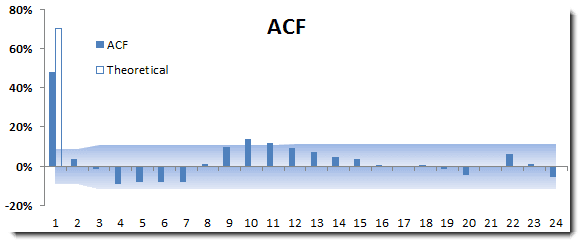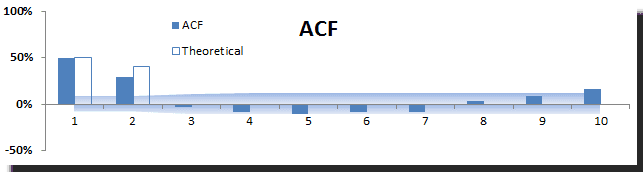INTUITION

1. The MA has a finite memory of size q
2. The ACF plot shows the memory size requirement of the model
3. An ARMA model with finite memory can be fully described using an MA type of model. The coefficients’ values of the MA model are the values of the auto-correlation function.

## Example 2 - AR(1) model

Next, let’s look at a simple auto-regressive (AR) model of order 1.

$$x_t=\phi _0+\phi_1x_{t-1}+a_t$$ $$(1-\phi_1L)x_t=\phi_0+a_t$$ $$(1-\phi_1L)(x_t-\mu )=a_t$$

Where

• $\mu=\frac{\phi_0}{1-\phi_1}$= long-run process (un-conditional) average
• $a_t\sim i.i.d\sim N(0,\sigma^2)$

Let’s compute the auto-correlation function of an AR(1) process:

$$(x_t-\mu)=y_t=\frac{a_t}{(1-\phi L)}$$

Assuming $\left \| \phi_1 \right \|< 1$, then AR(1) can be represented as an infinite MA model as below:

$$(x_t-\mu)=y_t=\frac{a_t}{(1-\phi_1 L)}=(1+\sum_{k=1}^{\infty }\phi_1^kL^k)a_t$$ $$\gamma _0=E\left [ x_t^2 \right ]=\left ( 1 +\sum_{k=1}^{\infty }\phi_1^{2k} \right )\sigma_a^2=\frac{\sigma_a^2}{1-\phi_1^2}$$ $$\rho_k=\frac{\phi_1^k\left ( 1+\sum_{j=1}^{\infty }\phi_1^{2j} \right )}{1+\sum_{j=1}^{\infty }\phi_1^{2j}} =\phi_1^k$$

The ACF plot for an AR-type of the process is infinite, but the $\rho_k$ decays exponentially.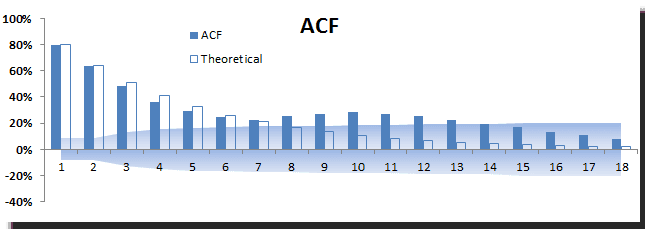## Example 3 - AR(p) model

Now, let’s get a bit more ambitious and look for an AR model with order p.

$$x_t=\phi_0+\phi_1x_{t-1}+\phi_2x_{t-2}+...+\phi_px_{t-p}+a_t$$ $$(1-\phi_1L-\phi_2L^2-...-\phi_pL^p)x_t=\phi_0+a_t$$ $$(1-\phi_1L-\phi_2L^2-...-\phi_pL^p)(x_t-\mu)=a_t$$ $$(1-r_1L)(1-r_2L)...(1-r_pL)(x_t-\mu)=a_t$$

Where

• $\mu=\frac{\phi_0}{1-\phi_1-\phi_2-...-\phi_p}=$ long-run unconditional process mean
• $r_1,r_1,...,r_p=$ characteristic roots of the AR(p) model

Let’s compute the auto-correlation function of an AR(p) process. Using partial-fraction decomposition, we break an AR(p) process into a set of p AR(1) process.

$$(x_t-\mu )=y_t=\frac{a_t}{(1-r_1L)(1-r_2L)...(1-r_pL)}=(\frac{\alpha_1}{(1-r_1L)}+\frac{\alpha_2}{(1-r_2L)}+...+\frac{\alpha_p}{(1-r_pL)})a_t$$

Let’s assume all characteristic roots fall outside the unit circle, and therefore the $\left \| r_k \right \|\prec 1$

$$(x_t-\mu)=y_t=\left ( 1+\alpha_1\sum_{k=1}^{\infty }r_1^kL^k+\alpha_2\sum_{k=1}^{\infty }r_2^kL^k+...+\alpha_p\sum_{k=1}^{\infty }r_p^kL^k\right )a_t$$ $$(x_t-\mu)=y_t=\left ( 1+\sum_{k=1}^{\infty }\psi ^kL^k\right )a_t$$ $$\psi ^k=\sum_{j=1}^{p}\alpha_jr_j^k$$ $$\gamma_0=\left ( 1+\sum_{j=1}^{p}\psi _j^2 \right )\sigma_a^2$$ $$\rho_k=\frac{\gamma _k}{\gamma_0}=\frac{\psi _k+\sum_{j=1}^{\infty }\psi_j\psi_{j+k}]}{1+\sum_{j=1}^{\infty}\psi_j^2}$$

This ACF plot is also infinite, but the actual shape can follow different patterns.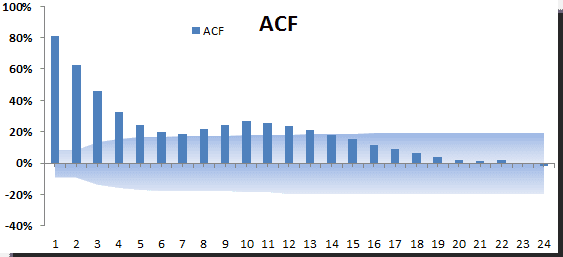INTUITION TWO:

1. An AR process can be represented by an infinite MA process
2. The AR has infinite memory, but the effect diminishes over time
3. Exponential smoothing functions are special cases of an AR process, and they also possess infinite memory

## Example 4 - ARMA(p,q) model

By now, we see what the ACF plot of a pure MA and AR process looks like, but what about a mixture of the two models?

Question: why do we need to consider a mixture model like ARMA, since we can represent any model as an MA or an AR model? Answer: we are trying to reduce the memory requirement and the complexity of the process by superimposing the two models.

$$x_t=\phi_0+\phi_1x_{t-1}+\phi_2x_{t-2}+...+\phi_px_{t-p}+a_t+\theta_1a_{t-1}+\theta_2a_{t-2}+...+\theta_qa_{t-q}$$ $$(1-\phi_1L-\phi_2L^2-...-\phi_pL^p)x_t=\phi_0+(1+\theta_1L+\theta_2L^2+...+\theta_qL^q)a_t$$ $$(1-\phi_1L-\phi_2L^2-...-\phi_pL^p)(x_t-\mu)=(1+\theta_1L+\theta_2L^2+...+\theta_qL^q)a_t$$ $$(1-r_1L)(1-r_2L)...(1-r_pL)(x_t-\mu)=(1+\theta_1L+\theta_2L^2+...+\theta_qL^q)a_t$$ $$(x_t-\mu)=y_t=\frac{(1+\theta_1L+\theta_2L^2+...+\theta_qL^q)}{(1-r_1L)(1-r_2L)...(1-r_pL)}a_t$$ $$y_t=\left ( \frac{\alpha_1}{(1-r_1L)}+\frac{\alpha_2}{(1-r_2L)}+...+\frac{\alpha_p}{(1-r_pL)} \right )\times(1+\theta_1L+\theta_2L^2+...+\theta_qL^q)a_t$$ $$y_t=(1+\psi_1L+\psi_2L^2+\psi_3L^3+...)\times(1+\theta_1L+\theta_2L^2+...+\theta_qL^q)a_t$$ $$y_t=(1+\omega _1L+\omega_2L^2+...+\omega_qL^q+...)a_t$$

Where

• $\mu=\frac{\phi_0}{1-\phi_1}=$ long-run conditional process mean (from the AR component
• $r_1,r_1,...,r_p=$ inverse of the characteristic roots of the AR(p) model
• $\psi ^k=\sum_{j=1}^{p}\alpha_jr_j^k$
• $\omega_1=\theta_1+\psi_1$
• $\omega_2=\theta_2+\theta_1\times\psi_1+\psi_2$
• $\omega_3=\theta_3+\theta_2\times\psi_1+\theta_1\times\psi_2+\psi_3$
• $\omega_q=\theta_q+\sum_{j=1}^{q-1}\theta_{q-j}\times\psi_j+\psi_q$
• $\omega_{q+k}=\sum_{j=0}^{q-1}\theta_{q-j}\times\psi_{j+k}+\psi_{q+k}$
• $\gamma _o=\left ( 1+\sum_{j=1}^{\infty}\omega_j^2 \right )\sigma_a^2$

Using the MA(q) auto-correlation formula, we can compute the ARMA(p,q) auto-correlation functions for their MA representation.

This is getting intense! Some of you might be wondering why we haven’t used VAR or a state-space representation to simplify the notations. I made a point to stay in the time domain, and avoided any new ideas or math tricks as they would not serve our intentions here: Implying the exact AR/MA order using the ACF values by themselves, which is anything but precise.

1. Intuition: The ACF values can be thought of as the coefficient values of the equivalent MA model.
2. Intuition: The conditional variance has no barrier (effect) on the auto-correlation calculations.
3. Intuition: The long-run mean $\mu$ also does not have any barrier (effect) on the auto-correlations.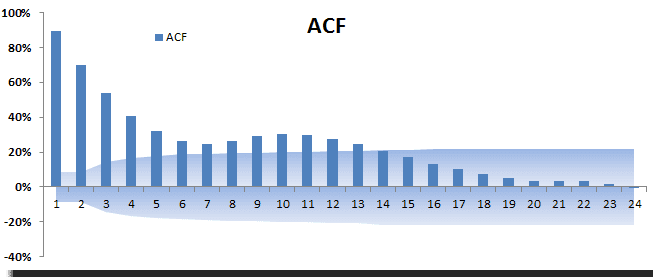## Partial Auto-correlation function (PACF)

By now, we have seen that identifying the model order (MA or the AR) is non-trivial for non-simple cases, so we need another tool – the partial autocorrelation function (PACF).

The partial autocorrelation function (PACF) plays an important role in data analysis aimed at identifying the extent of the lag in an autoregressive model. The use of this function was introduced as part of the Box-Jenkins approach to time series modeling, whereby one could determine the appropriate lags p in an AR(p) model or in an extended ARIMA(p,d,q) model by plotting the partial autocorrelation functions.

Simply put, the PACF for lag k is the regression coefficient for the kth term, as shown below:

$$x_t=b_{1,1}x_{t-1}+\varepsilon_{1,t}$$ $$x_t=b_{1,2}x_{t-1}+b_{2,2}x_{t-2}+\varepsilon_{2,t}$$ $$x_t=b_{1,3}x_{t-1}+b_{2,3}x_{t-2}+b_{3,3}x_{t-3}+\varepsilon_{2,t}$$ $$...$$ $$x_t=b_{1,k}x_{t-1}+b_{2,k}x_{t-2}+...+b_{k,k}x_{t-k}+\varepsilon_{k,t}$$

The PACF assumes the underlying model is an AR(k) and uses multiple regressions to compute the last regression coefficient.

Please note that $PACF(k)=b_{k,k}$, and that $PACF^{b_{k,k}\neq b_{k,k+1}\neq b_{k,k+2}\neq b_{k,k+2}\neq b_{k,k+2}...}$

Quick intuition: the PACF values can be thought of (roughly speaking) as the coefficient values of the equivalent AR model.

How is the PACF helpful to us? Assuming we have an AR(p) process, then the PACF will have significant values for the first p lags, and will drop to zero afterward.

What about the MA process? The MA process has non-zero PACF values for a (theoretically) infinite number of lags.

## Example 5: MA(1)

$$x_t-\mu=y_t=(1+\theta L)a_t$$ $$\frac{y_t}{(1+\theta L)}=a_t$$

Assuming$\left \| \theta \right \| \prec 1$ (invertible), the MA process can be represented as infinite AR:

$$(1-\theta L+\theta^2 L^2-...)y_t=a_t$$ $$y_t=a_t+\theta y_{t-1}-\theta^2 y_{t-2}+\theta^3 y_{t-3}+...+(-1)^{n+1}\theta^n y_{t-n}+...$$

The PACF of an MA is expected to have significant values to a large number of lags.

How about MA(q)? The same conclusion follows through here so that the MA(q) can be inverted to an infinite AR sequence.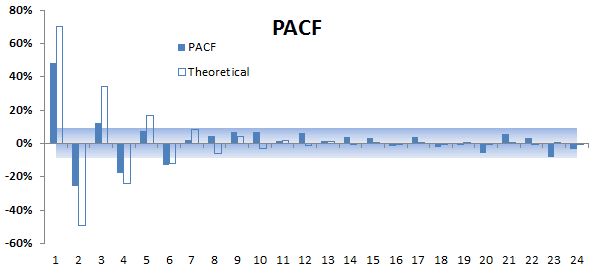## Conclusion

In this tutorial, we discussed the auto and partial autocorrelation functions and their role in identifying the order of the underlying ARMA process: ACF for the MA order and the PACF for the AR order.

Furthermore, we showed how more than one model can be used to generate the same ACF (and PACF) plots (i.e. correlogram).

With the exception of trivial cases, the process of identifying the proper model order is never clear-cut. The process demands that we entertain several candidate models, fit their parameters, validate the assumptions, compare their fit, and finally select the best one.